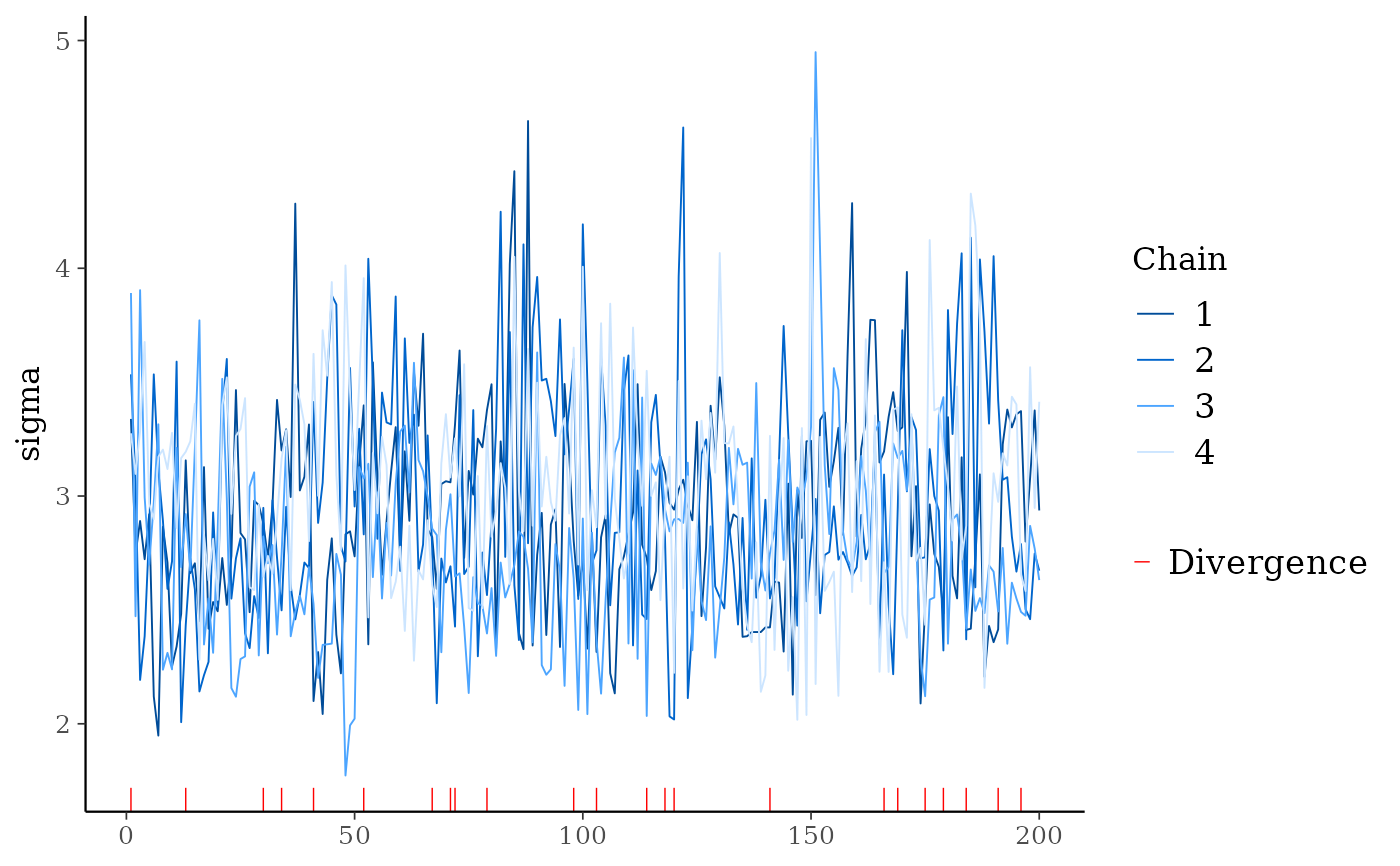Trace plot (or traceplot) of MCMC draws. See the Plot Descriptions section, below, for details.

mcmc_trace(x, pars = character(), regex_pars = character(),
transformations = list(), ..., facet_args = list(), n_warmup = 0,
iter1 = 0, window = NULL, size = NULL, np = NULL,
np_style = trace_style_np(), divergences = NULL)

mcmc_trace_highlight(x, pars = character(), regex_pars = character(),
transformations = list(), ..., facet_args = list(), n_warmup = 0,
window = NULL, size = NULL, alpha = 0.2, highlight = 1)

trace_style_np(div_color = "red", div_size = 0.25, div_alpha = 1)

mcmc_rank_overlay(x, pars = character(), regex_pars = character(),
transformations = list(), ..., n_bins = 20, ref_line = FALSE)

mcmc_rank_hist(x, pars = character(), regex_pars = character(),
transformations = list(), ..., facet_args = list(), n_bins = 20,
ref_line = FALSE)

mcmc_trace_data(x, pars = character(), regex_pars = character(),
transformations = list(), ..., highlight = NULL, n_warmup = 0,
iter1 = 0)

Arguments

x A 3-D array, matrix, list of matrices, or data frame of MCMC draws. The MCMC-overview page provides details on how to specify each these allowed inputs. It is also possible to use an object with an as.array() method that returns the same kind of 3-D array described on the MCMC-overview page. An optional character vector of parameter names. If neither pars nor regex_pars is specified then the default is to use all parameters. As of version 1.7.0, bayesplot also supports 'tidy' parameter selection by specifying pars = vars(...), where ... is specified the same way as in dplyr::select(...) and similar functions. Examples of using pars in this way can be found on the Tidy parameter selection page. An optional regular expression to use for parameter selection. Can be specified instead of pars or in addition to pars. When using pars for tidy parameter selection, the regex_pars argument is ignored since select helpers perform a similar function. Optionally, transformations to apply to parameters before plotting. If transformations is a function or a single string naming a function then that function will be used to transform all parameters. To apply transformations to particular parameters, the transformations argument can be a named list with length equal to the number of parameters to be transformed. Currently only univariate transformations of scalar parameters can be specified (multivariate transformations will be implemented in a future release). If transformations is a list, the name of each list element should be a parameter name and the content of each list element should be a function (or any item to match as a function via match.fun(), e.g. a string naming a function). If a function is specified by its name as a string (e.g. "log"), then it can be used to construct a new parameter label for the appropriate parameter (e.g. "log(sigma)"). If a function itself is specified (e.g. log or function(x) log(x)) then "t" is used in the new parameter label to indicate that the parameter is transformed (e.g. "t(sigma)"). Note: due to partial argument matching transformations can be abbreviated for convenience in interactive use (e.g., transform). Currently ignored. A named list of arguments (other than facets) passed to ggplot2::facet_wrap() or ggplot2::facet_grid() to control faceting. An integer; the number of warmup iterations included in x. The default is n_warmup = 0, i.e. to assume no warmup iterations are included. If n_warmup > 0 then the background for iterations 1:n_warmup is shaded gray. An integer; the iteration number of the first included draw (default is 0). This can be used to make it more obvious that the warmup iterations have been discarded from the traceplot. It cannot be specified if n_warmup is also set to a positive value. An integer vector of length two specifying the limits of a range of iterations to display. An optional value to override the default line size for mcmc_trace() or the default point size for mcmc_trace_highlight(). For models fit using NUTS (more generally, any symplectic integrator), an optional data frame providing NUTS diagnostic information. The data frame should be the object returned by nuts_params() or one with the same structure. If np is specified then tick marks are added to the bottom of the trace plot indicating within which iterations there was a divergence (if there were any). See the end of the Examples section, below. A call to the trace_style_np() helper function to specify arguments controlling the appearance of tick marks representing divergences (if the np argument is specified). Deprecated. Use the np argument instead. For mcmc_trace_highlight(), passed to ggplot2::geom_point() to control the transparency of the points for the chains not highlighted. For mcmc_trace_highlight(), an integer specifying one of the chains that will be more visible than the others in the plot. Optional arguments to the trace_style_np() helper function that are eventually passed to ggplot2::geom_rug() if the np argument is also specified. They control the color, size, and transparency specifications for showing divergences in the plot. The default values are displayed in the Usage section above. For the rank plots, the number of bins to use for the histogram of rank-normalized MCMC samples. Defaults to 20. For the rank plots, whether to draw a horizontal line at the average number of ranks per bin. Defaults to FALSE.

Value

The plotting functions return a ggplot object that can be further customized using the ggplot2 package. The functions with suffix _data() return the data that would have been drawn by the plotting function.

mcmc_trace_data() returns the data for the trace and rank plots in the same data frame.

Plot Descriptions

mcmc_trace()

Standard trace plots of MCMC draws. For models fit using NUTS, the np argument can be used to also show divergences on the trace plot.

mcmc_trace_highlight()

Traces are plotted using points rather than lines and the opacity of all chains but one (specified by the highlight argument) is reduced.

mcmc_rank_hist()

Whereas traditional trace plots visualize how the chains mix over the course of sampling, rank histograms visualize how the values from the chains mix together in terms of ranking. An ideal plot would show the rankings mixing or overlapping in a uniform distribution. See Vehtari et al. (2019) for details.

mcmc_rank_overlay()

Ranks from mcmc_rank_hist() are plotted using overlaid lines in a single panel.

Vehtari, A., Gelman, A., Simpson, D., Carpenter, B., Bürkner, P. (2019). Rank-normalization, folding, and localization: An improved R-hat for assessing convergence of MCMC. arXiv preprint.

Other MCMC: MCMC-combos, MCMC-diagnostics, MCMC-distributions, MCMC-intervals, MCMC-nuts, MCMC-overview, MCMC-parcoord, MCMC-recover, MCMC-scatterplots

Examples

# some parameter draws to use for demonstration
x <- example_mcmc_draws(chains = 4, params = 6)
dim(x)#>  250   4   6dimnames(x)#> $Iteration #> NULL #> #>$Chain
#>  "chain:1" "chain:2" "chain:3" "chain:4"
#>
#> \$Parameter
#>  "alpha"   "sigma"   "beta" "beta" "beta" "beta"
#>
# trace plots of the betas
color_scheme_set("viridis")
mcmc_trace(x, regex_pars = "beta")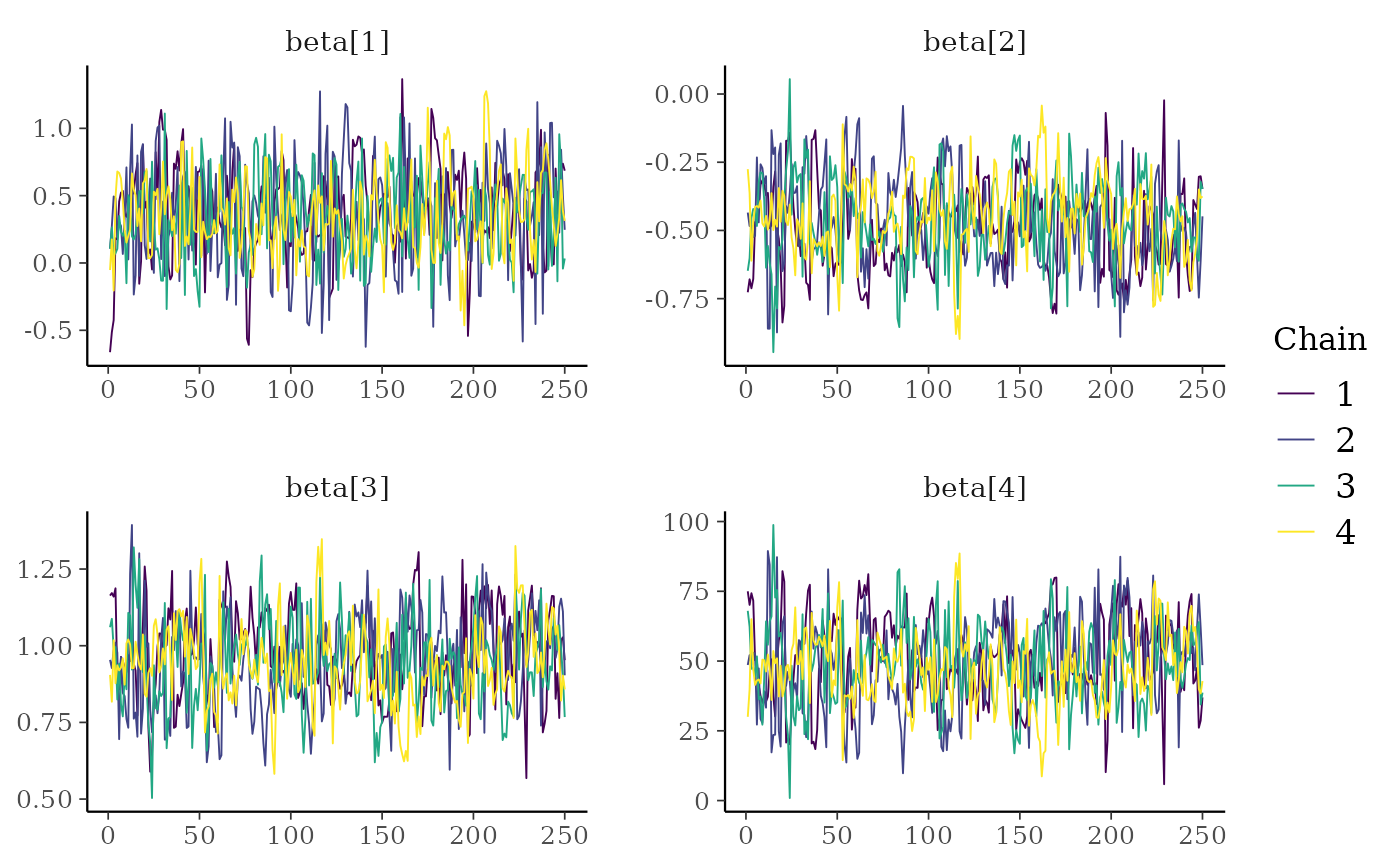color_scheme_set("viridisA")
mcmc_trace(x, regex_pars = "beta")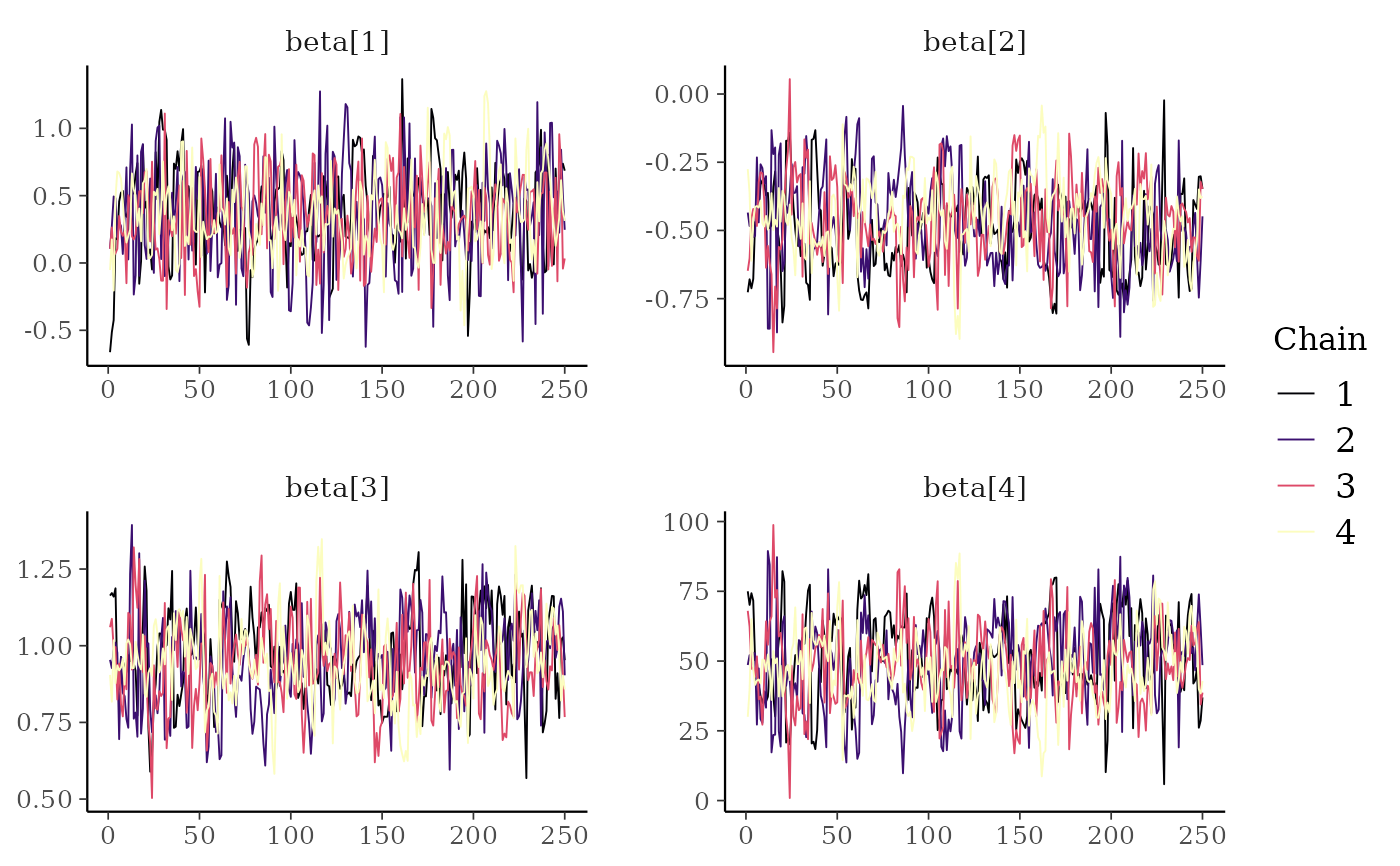color_scheme_set("viridisC")
mcmc_trace(x, regex_pars = "beta")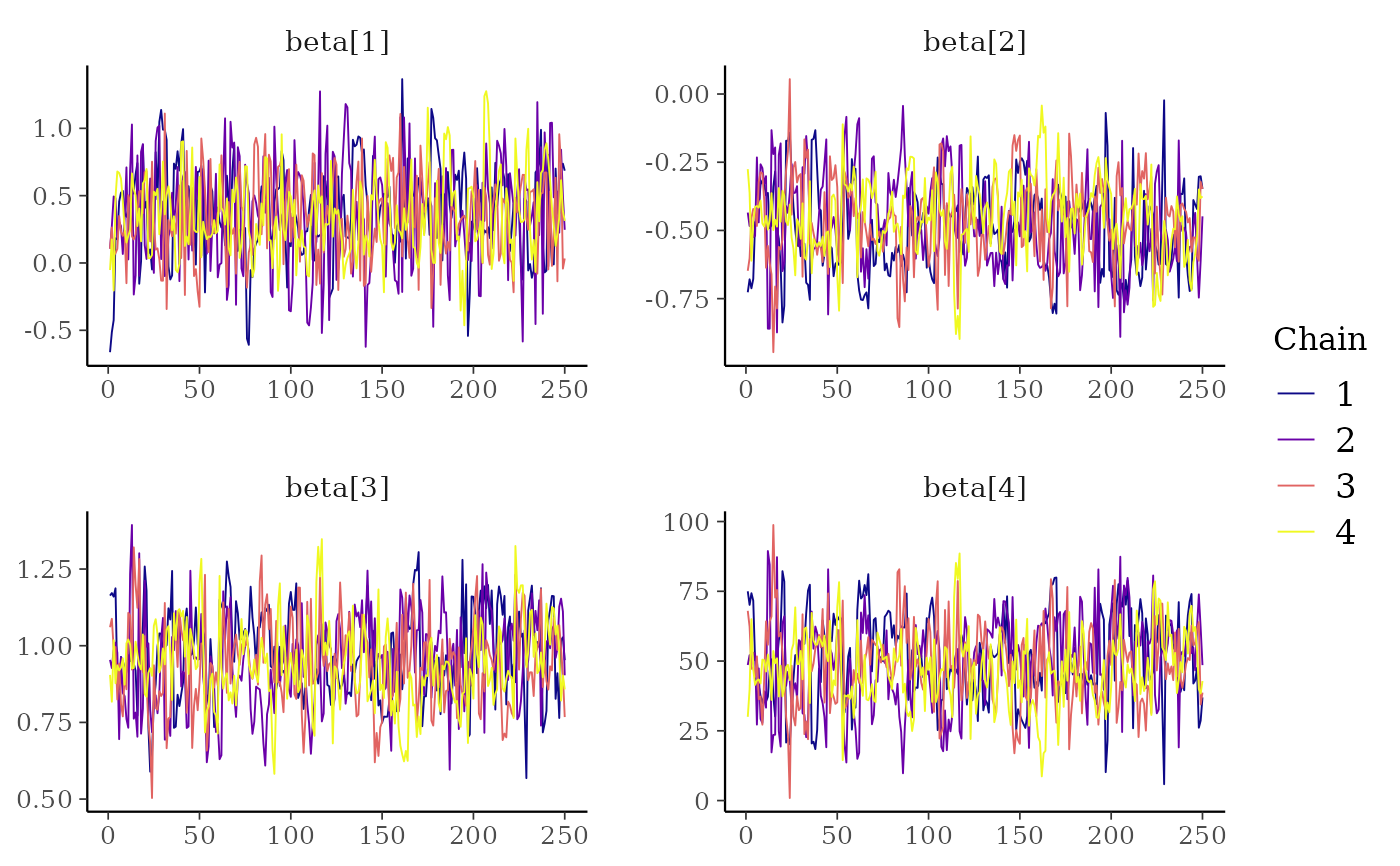# mix color schemes
color_scheme_set("mix-blue-red")
mcmc_trace(x, regex_pars = "beta")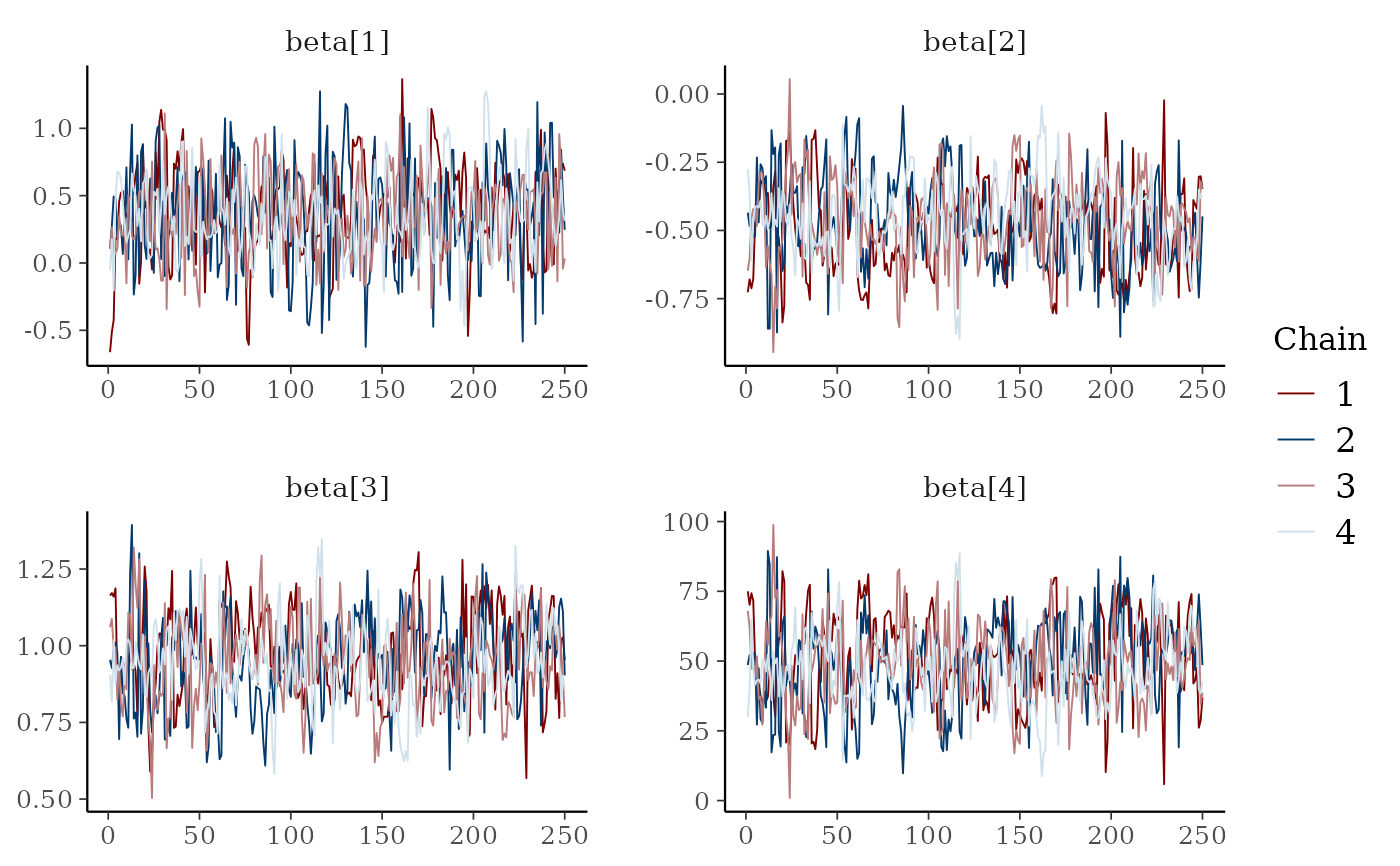# use traditional ggplot discrete color scale
mcmc_trace(x, pars = c("alpha", "sigma")) +
ggplot2::scale_color_discrete()#> Scale for 'colour' is already present. Adding another scale for 'colour',
#> which will replace the existing scale.# zoom in on a window of iterations, increase line size,
# add tick marks, move legend to the top, add gray background
color_scheme_set("viridisA")
mcmc_trace(x[,, 1:4], window = c(100, 130), size = 1) +
panel_bg(fill = "gray90", color = NA) +
legend_move("top")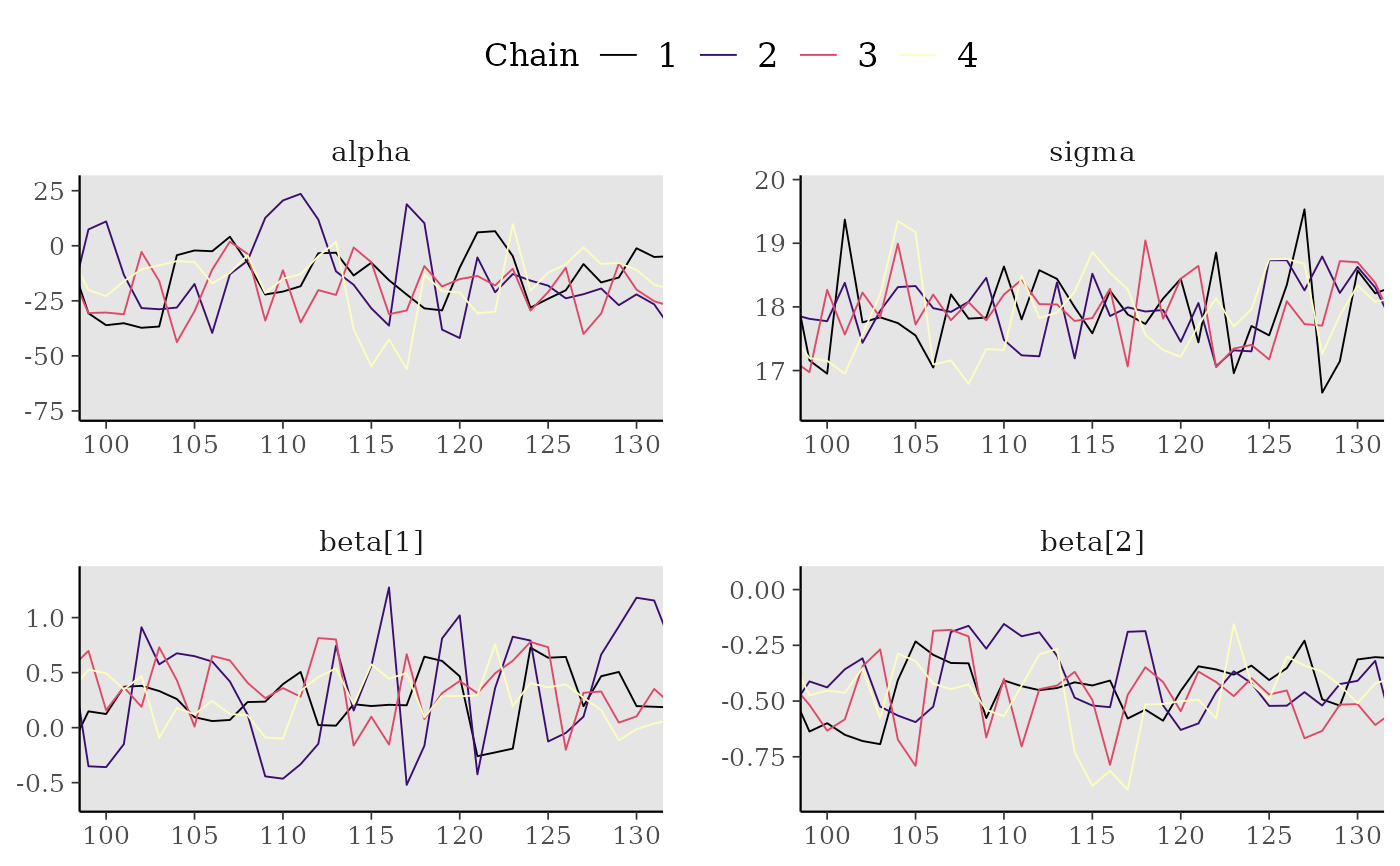# Rank-normalized histogram plots. Instead of showing how chains mix over
# time, look at how the ranking of MCMC samples mixed between chains.
color_scheme_set("viridisE")
mcmc_rank_hist(x, "alpha")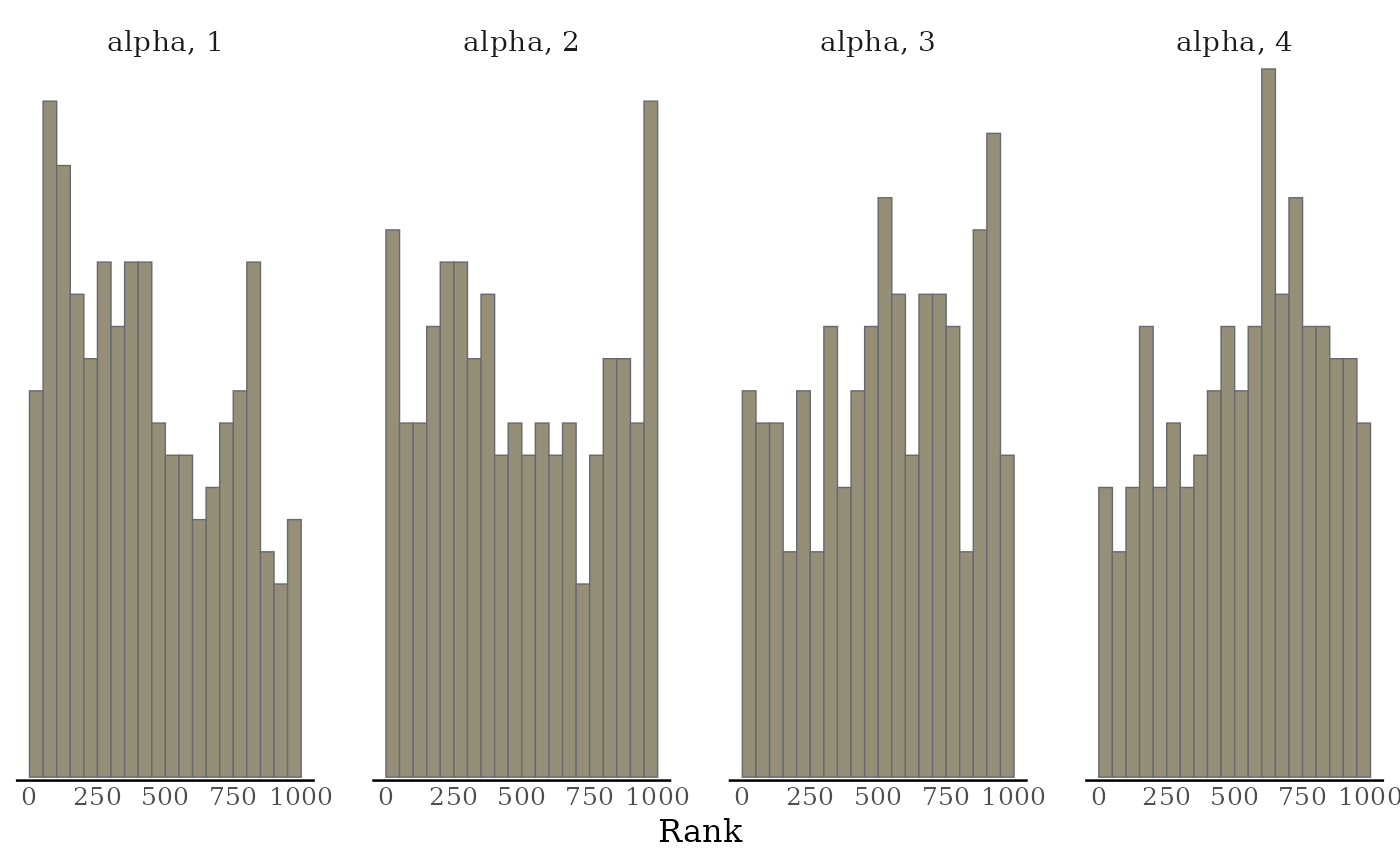mcmc_rank_hist(x, pars = c("alpha", "sigma"), ref_line = TRUE)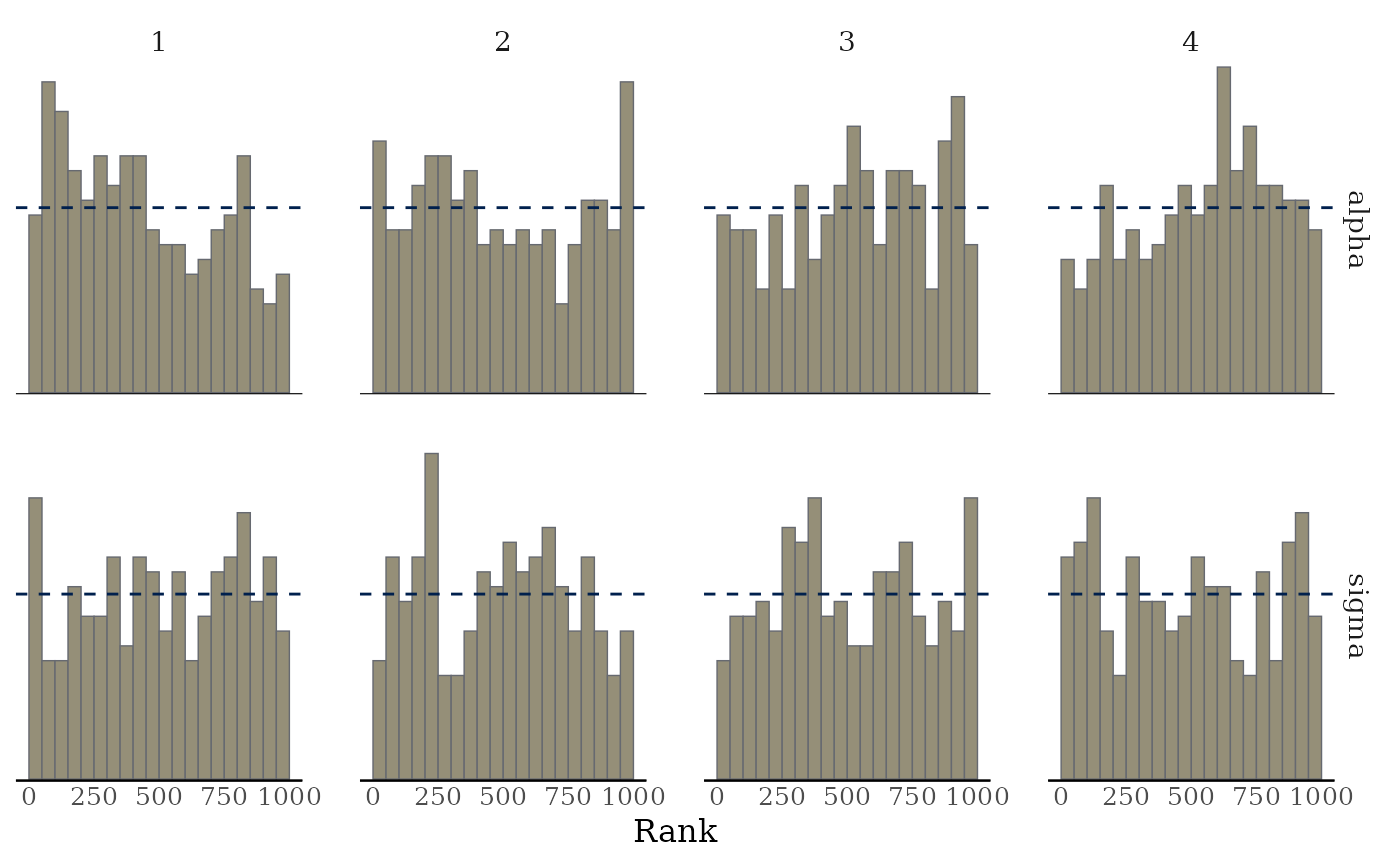mcmc_rank_overlay(x, "alpha")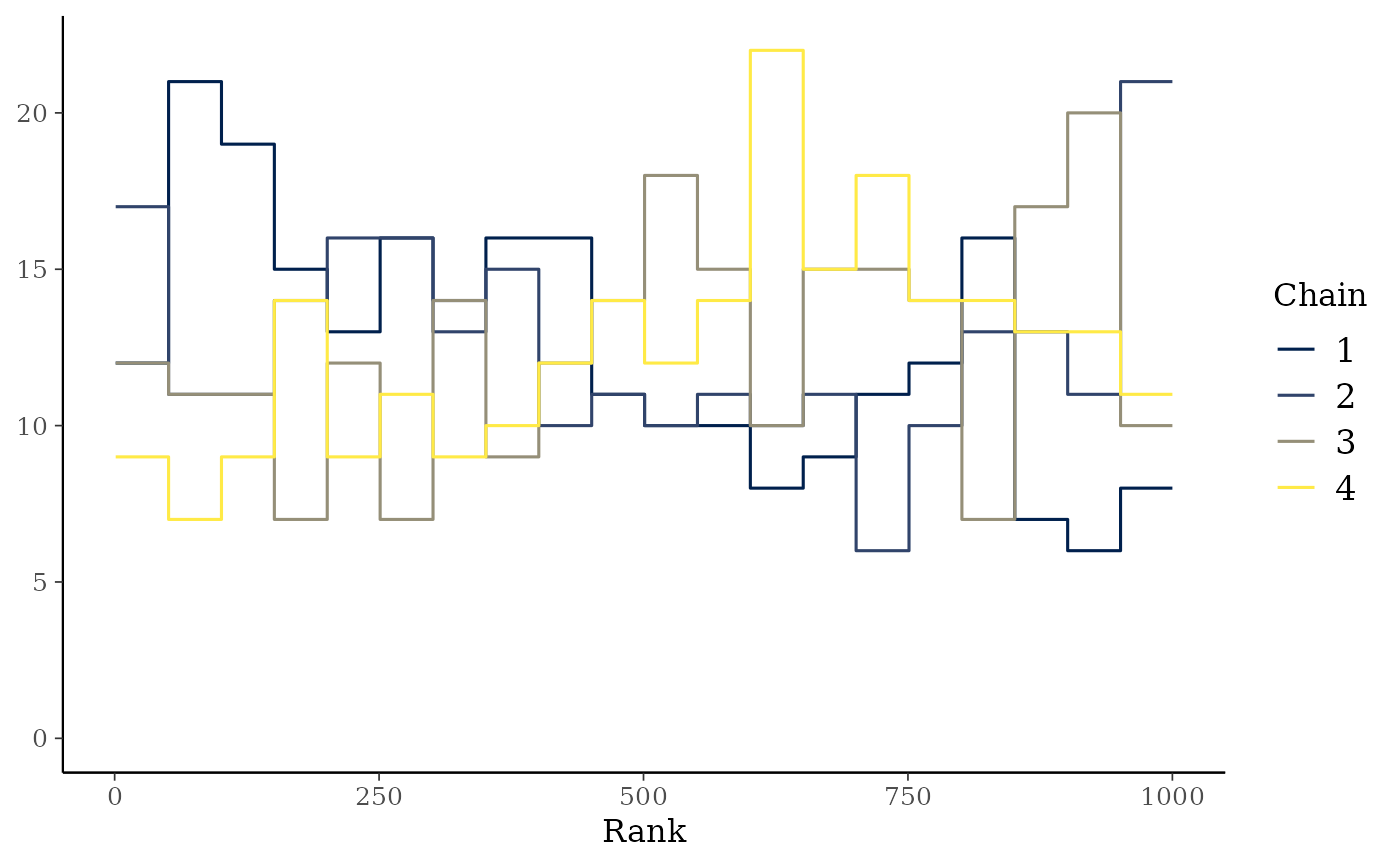# parse facet label text
color_scheme_set("purple")
p <- mcmc_trace(
x,
regex_pars = "beta\$[1,3]\$",
facet_args = list(labeller = ggplot2::label_parsed)
)
p + facet_text(size = 15)# mark first 100 draws as warmup
mcmc_trace(x, n_warmup = 100)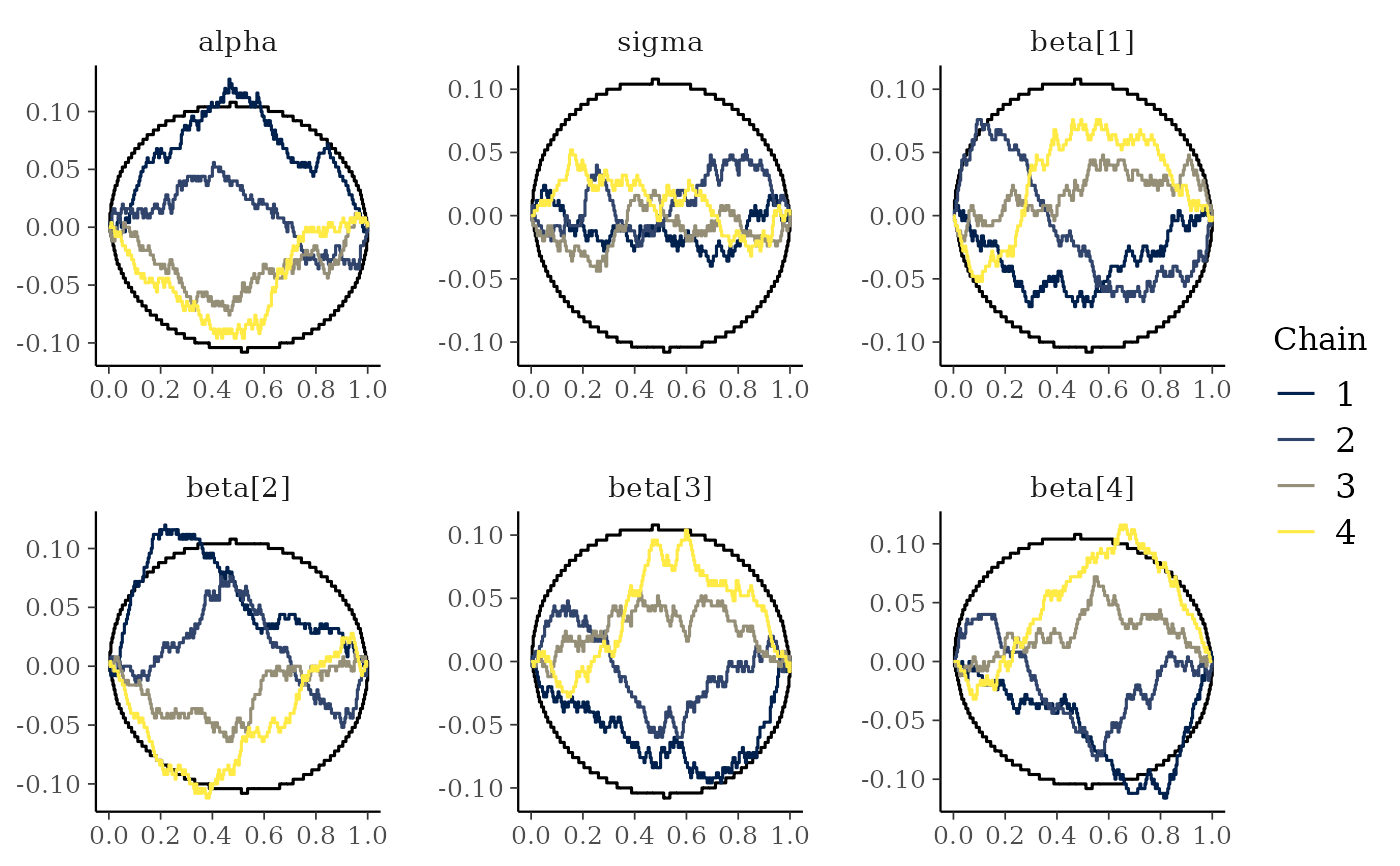# plot as points, highlighting chain 2
color_scheme_set("brightblue")
mcmc_trace_highlight(x, pars = "sigma", highlight = 2, size = 2)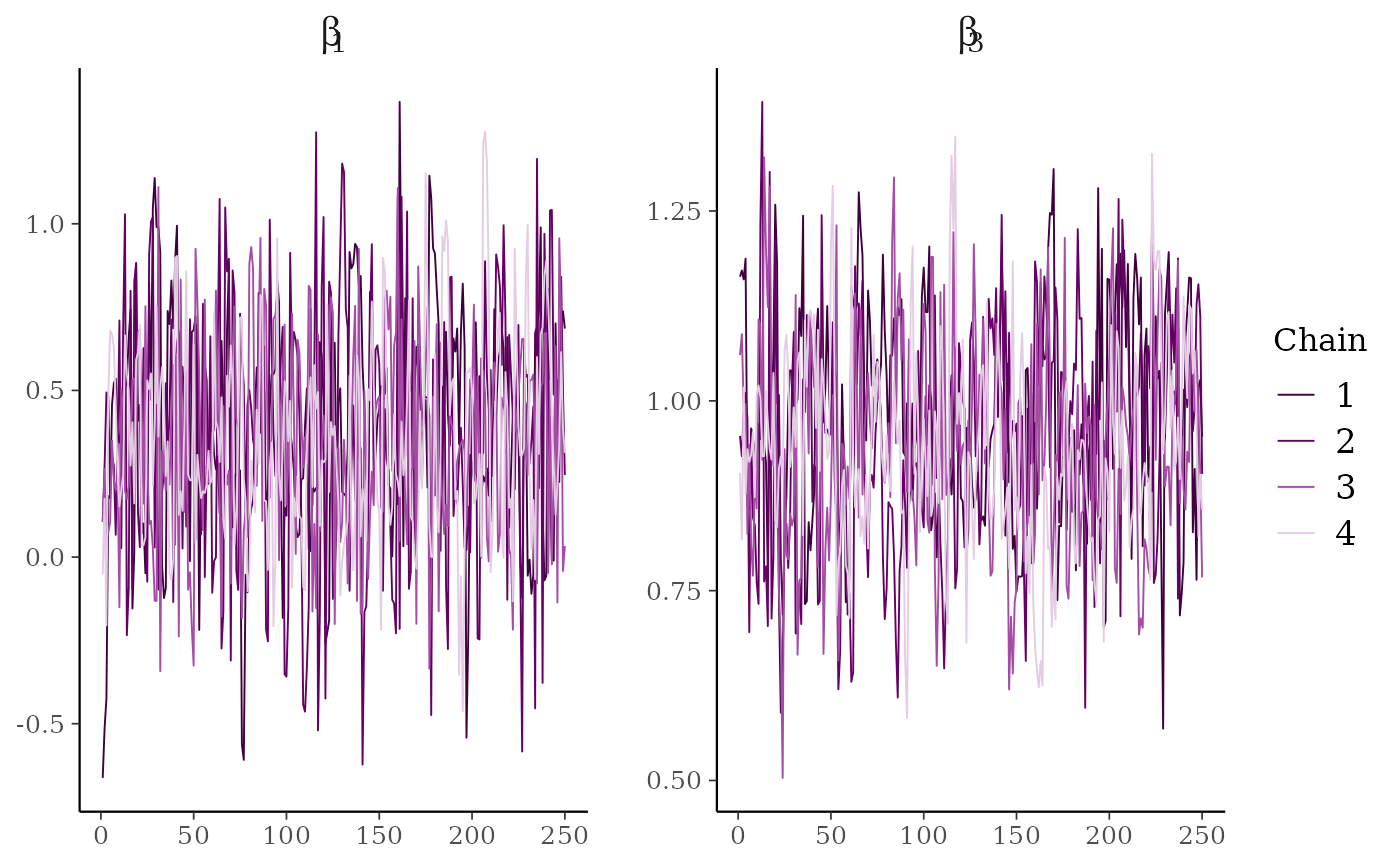# for models fit using HMC/NUTS divergences can be displayed in the trace plot
library("rstanarm")
fit <- stan_glm(mpg ~ ., data = mtcars, refresh = 0,
# next line to keep example fast and also ensure we get some divergences
prior = hs(), iter = 400, adapt_delta = 0.8)#> Warning: There were 23 divergent transitions after warmup. Increasing adapt_delta above 0.8 may help. See
#> http://mc-stan.org/misc/warnings.html#divergent-transitions-after-warmup#> Warning: Examine the pairs() plot to diagnose sampling problems#> Warning: Bulk Effective Samples Size (ESS) is too low, indicating posterior means and medians may be unreliable.
#> Running the chains for more iterations may help. See
#> http://mc-stan.org/misc/warnings.html#bulk-ess#> Warning: Tail Effective Samples Size (ESS) is too low, indicatingposterior variances and tail quantiles may be unreliable.
#> Running the chains for more iterations may help. See
#> http://mc-stan.org/misc/warnings.html#tail-ess
# extract draws using as.array (instead of as.matrix) to keep
# chains separate for trace plot
posterior <- as.array(fit)

# for stanfit and stanreg objects use nuts_params() to get the divergences
mcmc_trace(posterior, pars = "sigma", np = nuts_params(fit))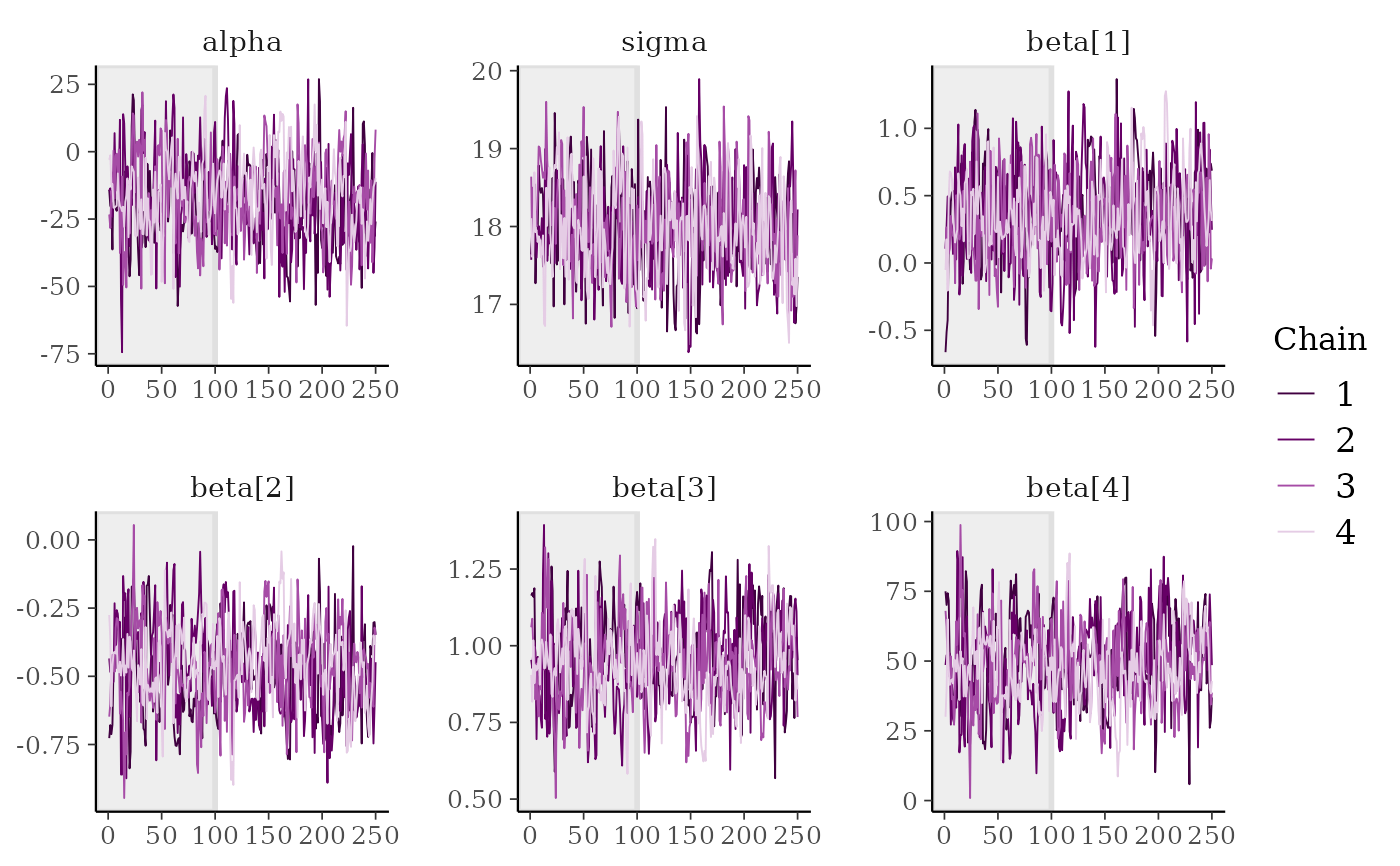color_scheme_set("viridis")
mcmc_trace(
posterior,
pars = c("wt", "sigma"),
size = 0.5,
facet_args = list(nrow = 2),
np = nuts_params(fit),
np_style = trace_style_np(div_color = "black", div_size = 0.5)
)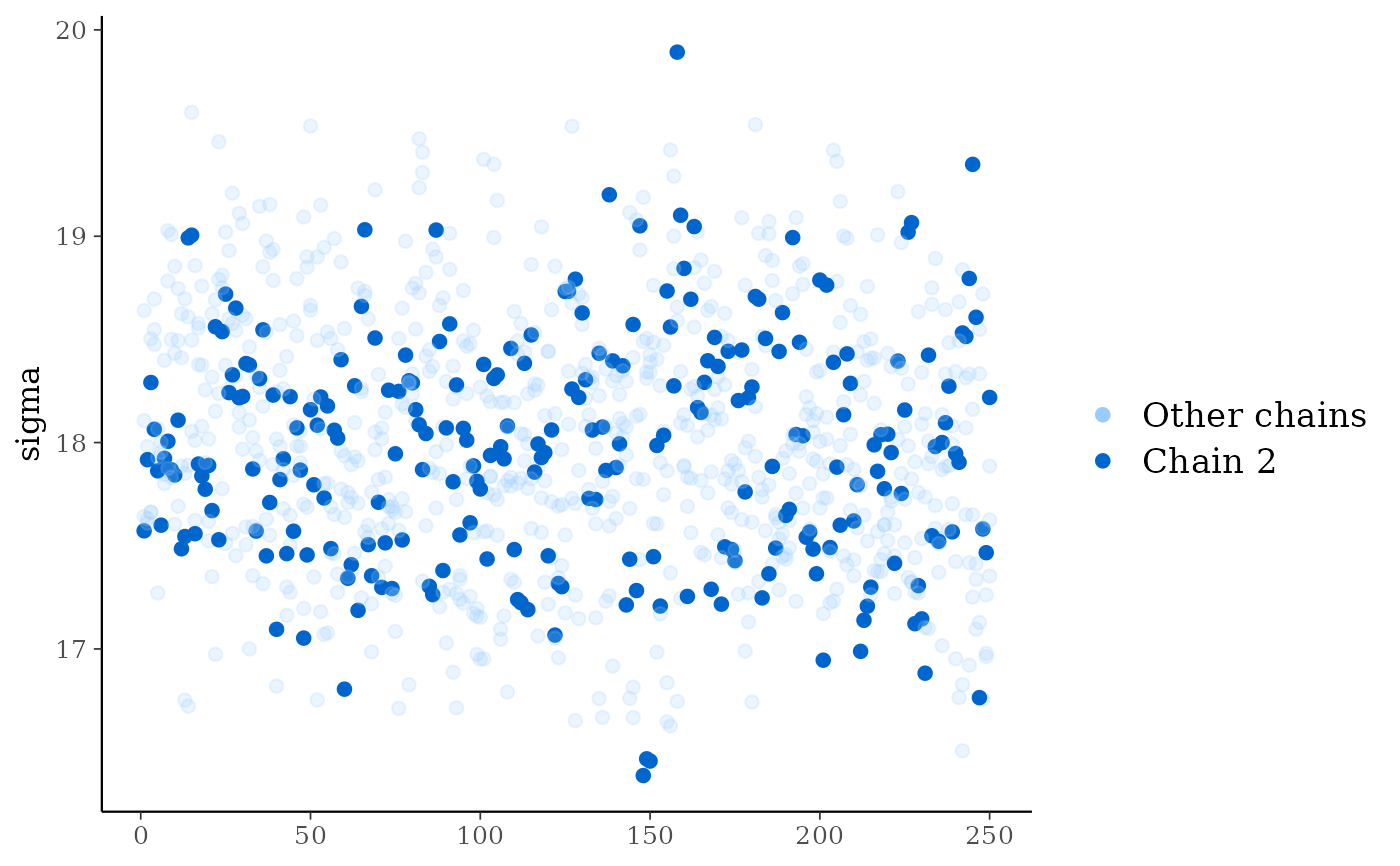mcmc_trace(
posterior,
pars = c("wt", "sigma"),
size = 0.8,
facet_args = list(nrow = 2),
divergences = nuts_params(fit),
div_color = "black"
)#> Warning: The 'divergences' argument is deprecated and will be removed in a future release. Use the 'np' argument instead.#> Warning: The following arguments were unrecognized and ignored: div_color